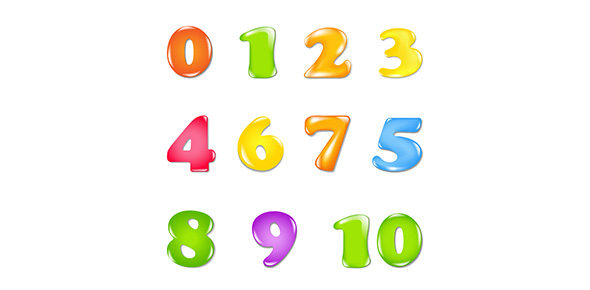# Comparing & Ordering Decimals

4 Questions | Total Attempts: 39SettingsThis is a quiz that tests students' ability to determine the relative sizes of numbers of up to 7 digits.

Related Topics
• 1.
Check all of the numbers that are LARGER than 487,212
• A.

299,889

• B.

1,001,203

• C.

76,581

• D.

478,203

• E.

519,002

• 2.
73,176,518      8,639,840                            Which symbol best replaces the  in the equation above?
• A.

>

• B.

• C.

=

• D.

+

• 3.
Replace the blank with greater than, less than, or equal to.  389,514 is ______________  1,214,319.
• 4.
Why is 59,412 larger than 9,416?
• A.

They both have a 9 in the thousands place.

• B.

Each number has the same number of digits.

• C.

The first number has more digits than the second number.

• D.

There are no 2s in the last number, but there is one in the first.